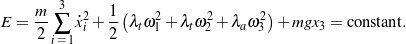# The Lagrange top

Referring to Figure 1 below, the Lagrange top is an axisymmetric rigid body whose point of contact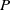with a horizontal surface is fixed. Such an object is referred to as the Lagrange top because Lagrange considered the dynamics of this body in his Mécanique Analytique (see ).

## Equations of motion

For the Lagrange top illustrated in Figure 1, we take its mass to beand assume the fixed contact pointis directly beneath the top’s mass center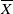such that the relative position vector foris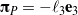, where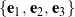is the top’s corotational basis. We may track the location of the top’s mass center using a set of Cartesian coordinates,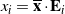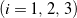, and therefore the linear momentum of the top is given by, where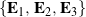represents the space-fixed basis. The orientation of the top may be described by, say, a 3-1-3 set of Euler angles: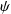,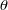, and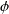.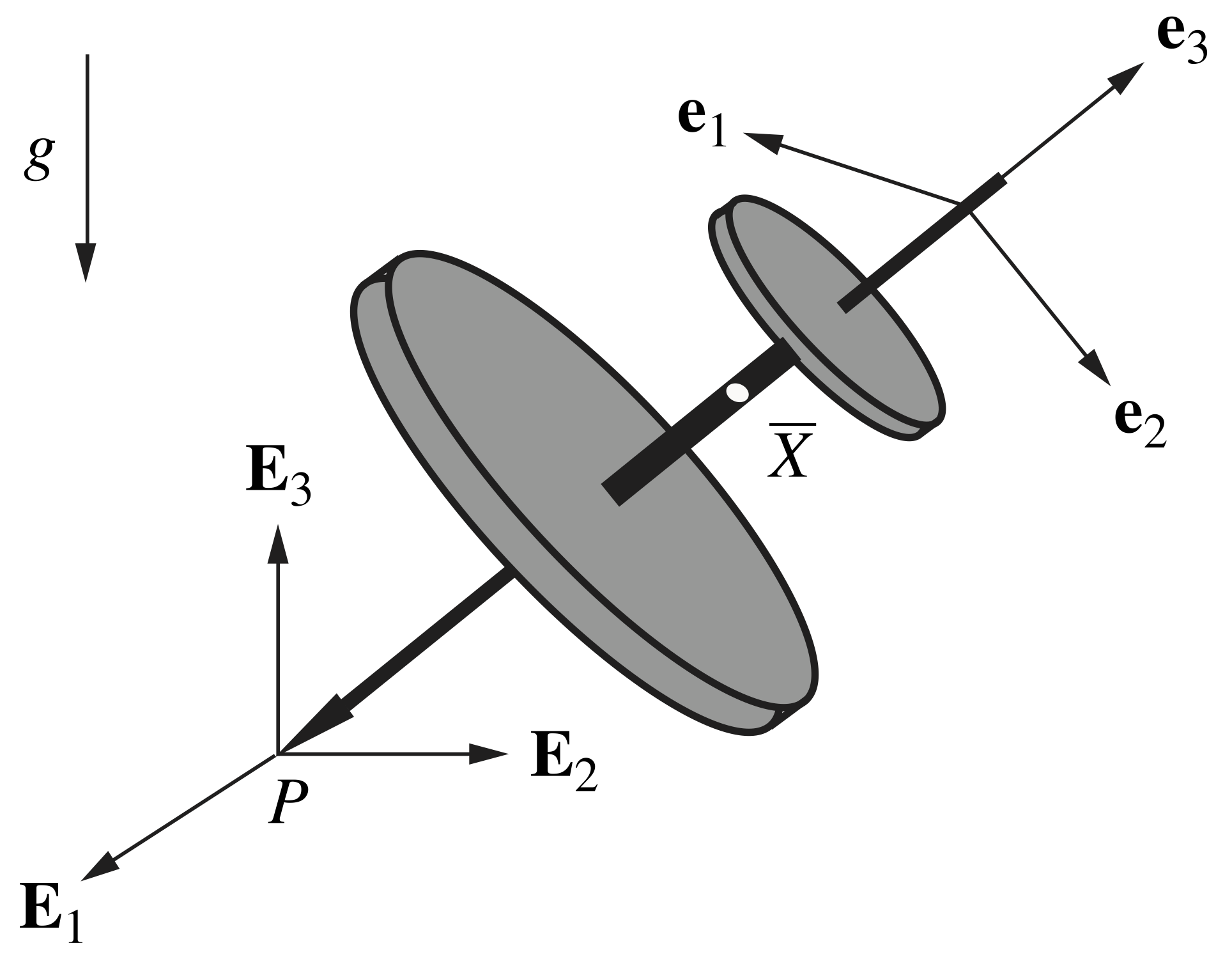Figure 1. Schematic of the Lagrange top illustrating the alignment of the corotational basis .

The Lagrange top’s longitudinal axis is assumed to be an axis of symmetry (i.e., the body is symmetric about thedirection), and thus the top’s principal moments of inertiaand. We may then express the angular momentum of the top about its center of mass as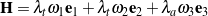, where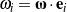are the corotational components of angular velocity that, for a 3-1-3 set of Euler angles, are related to,, andand their rates of change as follows:

(1)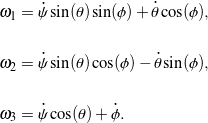The Lagrange top is subject to three integrable (holonomic) constraints of the formthat arise from the fact that the contact pointis fixed, i.e., the velocity. These constraints relate the location of the mass center to the top’s orientation.

The equations of motion for the Lagrange top may be obtained by applying a balance of linear momentum and a balance of angular momentum with respect to the top’s center of mass: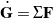and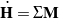, respectively, where the top’s weight and the reaction forces at the fixed contact pointcontribute to the net force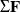and the net moment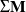about the mass center. The resulting differential equations from applying the balance laws can then be expressed in the first-order formfor numerical integration in MATLAB, where we define the state vector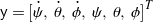:

(2)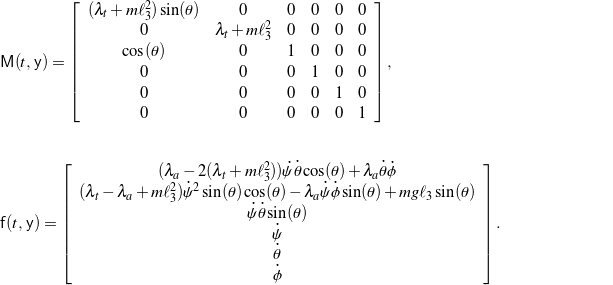## Simulation and animation

For an arbitrary choice of initial conditions for the Euler angles and their rates of change, numerical simulation shows that the Lagrange top generally behaves like a spherical pendulum, assuming the top is allowed to fall below the horizontal. A typical example of this pendulum-like behavior is animated in Figure 2.

However, it is possible for the Lagrange top to undergo a steady motion in which it precesses and spins at constant rates (that is,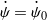and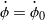, respectively, are constant) while at a fixed nutation angle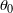under the following condition:

(3)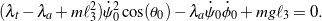An animation of a representative steady motion is shown in Figure 3.

The Lagrange top features several conservations. It can be shown that the top’s simulated motion conserves two components of the top’s angular momentum with respect to the fixed contact point:and. In addition, the top’s total mechanical energy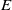is conserved:

(4)